# Pandas Interview Questions

## Overview

Pandas (Python Data Analysis Library) is a powerful tool for performing data manipulations, cleaning, and preparations with Python. Pandas utilize a unique data structure called a “DataFrame” that showcases 2-dimensional matrices that can better represent tabular data.

With its built-in functions and methods, Pandas allows easier data cleanup and manipulation by building on the arrays used in NumPy.

Below is a list of Pandas interview questions, sorted based on their difficulty.

## When Do You Use Pandas Interview Questions in Data Science?

Pandas is the go-to Python library for data manipulation from various sources, including file formats (.csv,.xslx,.json), structured query language (SQL) databases, and websites, especially when tabular data is involved.

Job titles that handle tabular data appreciate and expect a strong understanding of Pandas. See below for the job positions that require or value Pandas experience:

• Data Engineer
• Data Scientist
• Data Analyst
• Python SWE
• Machine Learning Engineer

## How Important is Pandas in Data Science?

Pandas plays an important role in helping clean and manage tabular data and allows data scientists to do more with less code. Moreover, because of the built-in modifications to primitive functions that come with the Pandas library, it typically runs through data faster than Python.

Because of this, at its core, Pandas is a tool that helps provide timely data analysis reports through ease of use and its sheer power.

For the following Pandas interview questions, we utilize Jupyter Notebook.

## Beginner Pandas Interview Questions

These easy Pandas interview questions can help you assess your level of understanding. The following questions are usually asked by the interviewer to identify the candidates that can proceed to the next step of the interview process.

### 1. What is Pandas?

Pandas is a Python library designed for connecting .csv, .xlsx, and other tabular-oriented data sources to Python, allowing you to create scripts that can help modify said DataFrames.

Although other libraries, such as NumPy arrays, are better suited for modifying purely numerical data, when a mix of data types is introduced, especially strings, Pandas provides the user with many control functions. It’s also useful for creating more presentable versions of data than arrays.

### 2. What data structures are primarily associated with Pandas and how do they work?

The Pandas library concerns itself with two primary data structures: the DataFrame and the series.

The DataFrame is a two-dimensional, mutable data structure with rows and columns as its axes. As mentioned earlier, Pandas’ DataFrame handles multiple data types, and each block (also referred to as a cell in a sheet) is identified by the data inside it, its row, and its column.

Meanwhile, the series is the one-dimensional counterpart to the DataFrame.

### 3. What are the key features of Pandas?

Pandas is a great library due to the following key features:

• Integrates with NumPy
• Easily presents tabular data
• Provides easy reshaping of tabular data
• Has useful functions for time series
• Easily fills in missing data
• Has memory efficiency
• Allows for easy merging, concatenating, and joining of two or more datasets
• Quickly aggregates data

### 4. How do you import a file from your computer to Pandas?

One of the most significant features in Pandas is the ability to equate a DataFrame variable to an entire .csv, .sql, and other tabular data files. How can you achieve this exactly?

The general syntax is typically similar to the following:

``````import pandas as pd
``````

For a more specific example, see the following:

``````import pandas as pd
``````

### 5. How do you print the information inside the DataFrame?

Building on the earlier code, you can import the DataFrame using Python’s built-in print() function. However, there are ways to manipulate the function’s behavior.

There is the default code that prints the DataFrame’s head (first five series) and the DataFrame’s tail (last five series):

``````import pandas as pd
df
``````

You can also print the data from index zero to n by using the head function (where n is the last index to print):

``````import pandas as pd
``````

You can do the same from the end using the tail function:

``````import pandas as pd
df.tail(n)
``````

Note: Leaving an object with an undeclared variable at the end of a Jupyter Notebook will print out the value of that variable.

### 6. How do you access a specific element inside a DataFrame?

To access a specific element inside a DataFrame, you can utilize the loc[] and at[] functions. The loc[] function can specify the column and the at[] function can determine the row of your specific element.

The syntax for this is as follows:

``````import pandas as pd
specificElement = myvar.loc[index].at['Row Name']
``````

Notice that the loc[] function takes in an integer whereas the at[] function takes in a string.

### 7. How do you print the entire DataFrame?

When you try to print a DataFrame using Pandas’ default setup, you may observe that Pandas will try to print only the head and the tail (meaning the first and last five series).

You can try to bypass this using the tail() and head() functions, wherein you pass the number of columns as the parameter. But you will soon realize that instead of displaying the entire DataFrame, Pandas will display the following: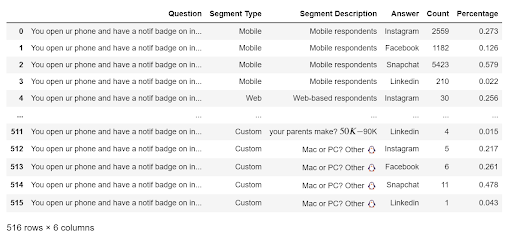This is not necessarily the result you want. As such, you can remove the print limitations using the following code:

``````pd.set_option("display.max_rows", None, "display.max_columns", None)
``````

All you need to do is print the DataFrame, and you should see the whole thing. Use the following code as an example:

``````import pandas as pd

pd.set_option("display.max_rows", None, "display.max_columns", None)
df
``````

## Intermediate Pandas Interview Questions

The following are some Intermediate Pandas interview questions:

### 1. What is the difference between merge and concatenate in Pandas?

Merge and concatenate are the most commonly used functions when fusing two datasets (a series or a DataFrame). Though they may seem the same, along with join() and append(), they all serve different purposes.

Merge is utilized for general use compared to concatenate. When you have two datasets that share information, merge is the best option.

Merge has two required parameters: the left and right datasets, and four variants: inner merge, outer merge, left merge, and right merge.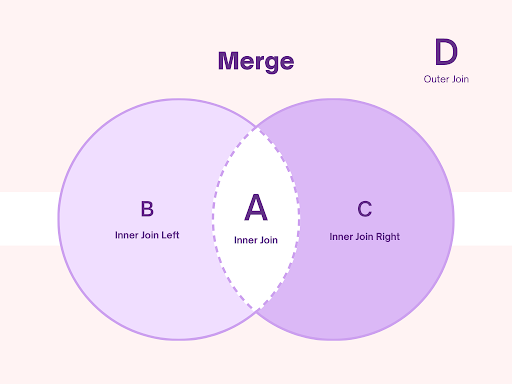The inner merges (including the left and right) put you at risk of losing data upon merging. However, the outer merge includes all data from both datasets.

Use the following code as an example, given a dataset:

``````import pandas as pd
dataFrameOne = pd.DataFrame({"Segment" : ['Mobile', 'Web', 'University'], "Answer" : ['Instagram', 'Facebook', 'Snapchat']})
dataFrameTwo = pd.DataFrame({"Count" : [2559, 32, 0], "Answer" : ['Instagram', 'Facebook', 'Snapchat']})
``````

With DataFrameOne:With DataFrameTwo: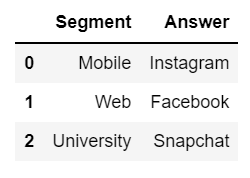You can use the following code to merge:

``````merged = pd.merge(dataFrameOne, dataFrameTwo)
``````

Which will give you: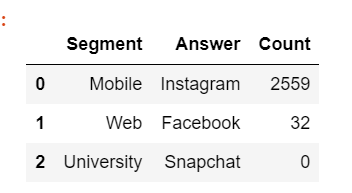As you can see, Pandas automatically merges rows and columns with the same value. However, if you use concatenate instead of merging datasets that contain columns of the same value and name, it will automatically stitch the two rows together.

Using the following code:

``````conc = pd.concat([dataFrameOne, dataFrameTwo], axis = "columns")
``````

You will get the following result: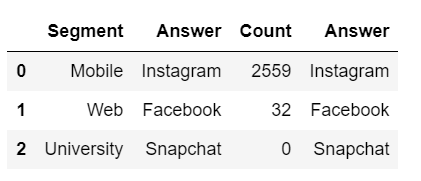Even though the value of the “Answer” columns is the same, it is added twice to the fused dataset due to the nature of concatenation.

### 2. How do you select specific data types to include or exclude in your DataFrame?

For Pandas, one function helps you include or exclude specific data types into a DataFrame.

The select_dtypes() function can help you include or exclude specific elements based on whether they are of the specified function inside the parameter.

For example, given the specific DataFrame:Using the following code:

``````df.select_dtypes(include='int64')
``````

You get the following output: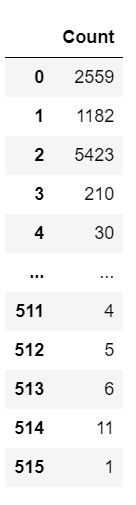This results in a DataFrame with only the columns of the datatype ‘int64’. If you use the exclude keyword argument instead of include, however, you get the following result: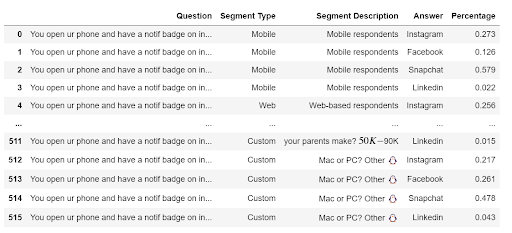Using the following code:

``````df.select_dtypes(exclude='int64')
``````

### 3. What is a Pivot Table? How Do You Create One?

A pivot table in Pandas is a valuable tool that allows you to summarize and aggregate data. To create a pivot table, use the pivot() function to help reshape and aggregate data into a compact form.

Given the previous DataFrame:You can create a pivot table that aggregates all the answers and their frequencies and links them to their specific demographic (using the segment description column) with the following code:

``````pvt = df.pivot_table('Count', index = 'Segment Description', columns = 'Answer')
``````

Which will result in this aggregated table: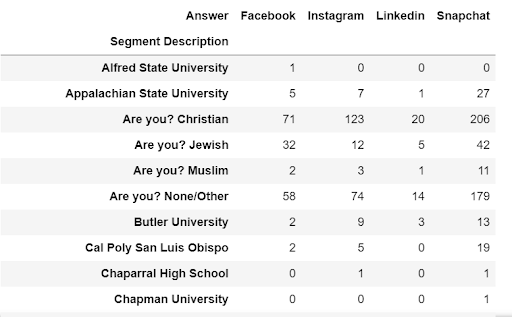As you can see, this table displays more insight into the answers per demographic and allows you to create assumptions at a glance. While pivot tables should not solely be the basis of your conclusion, they help generate a bird’s eye view of the data.

### 4. What is melt() and how do you use this function?

In Pandas, melt() is an integral tool for reshaping DataFrames from wide to long format. Most utilize melt() to transpose DataFrame data into a computer-readable form factor, allowing for easier transposition when involving other libraries aside from Pandas.

Below is our demonstration of how melt() works, given a dataset df: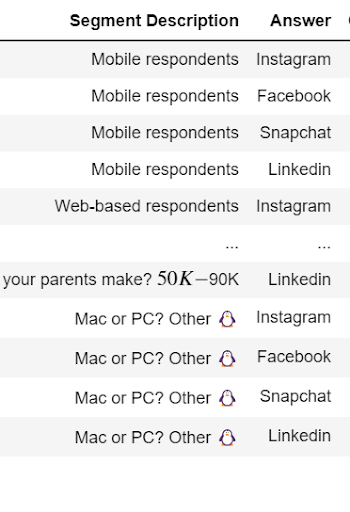You can use the general syntax of melt(), which is:

``````var = pd.melt(<DataFrame>, id_vars =[ '<identifier column>]', value_vars = ['<column to unpivot>'])
``````

And perform your own melt() using the following code:

``````melted = pd.melt(df, id_vars = ['Segment Description'], value_vars = ['Answer'])
melted
``````

This displays the following: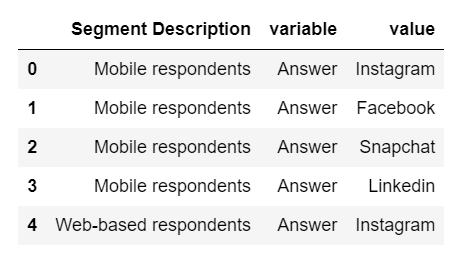### 5. How do you handle missing data in Pandas?

Missing data is a far too common problem data scientists, analysts, and engineers face daily. Thankfully, Pandas is a great tool for cleaning datasets and allowing you to eliminate or supplement missing data from your DataFrame.

One of the fundamental things to know about missing data is their Pandas representation. Typically, missing data in Pandas is represented by the NaN (not a number, floating type) and None (object, singleton) keywords.

To check for missing data, you can use the isnull() and notnull() functions. On the other hand, if you want to fill in missing data, you can use the following functions:

• `fillna()` allows you to place values to null objects, either by replacing all instances with a specific value or by using the method parameter to replace all null values with the last observed valid ones. This method is incredibly flexible for modifying missing values
• `replace()` allows you to replace all null values with a specific value
• `dropna()` allows you to drop values
• `interpolation()` allows you to change values with interpolation methods

## 6. What is groupby() and how can you use it effectively to create aggregations?

The groupby() function allows you to create clusters using a specific identifier. Given the previous DataFrame:You can use the groupby() function to group the DataFrame as per their answer using the following code:

``````gp = df.groupby(["Answer"]).sum()
``````

You can then view the first group by typing the following:

``````gp.head()
``````Or you can also type the following:

``````gp.sum()
``````

To get the following result: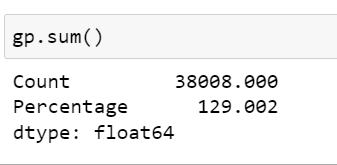### 1. CleanSpark: Electricity Pricing Model Take HomeYou are given a month’s electricity consumption data (kWh) in 15-minute intervals for a commercial facility located in San Diego, CA. These data can be read into a Pandas DataFrame using the pre-coded `_get_data` function in the cell below. Each interval is labeled with the time it began, and all intervals are 15 minutes long. Your task is to add logic to the two stubbed functions in the cell below module. The first method is called `energy_charge` and the second is called `demand_charge`.

An example pricing scheme (electricity tariff) is outlined below. Your functions will need to use the electricity consumption data and the pricing scheme to calculate the energy and demand charges. Please feel free to import any other third-party packages you think you will need. In addition, feel free to write as many extra helper functions as you need and include them in the cell below file as well.

For the succeeding Pandas interview questions, use the given dataset, df, to perform the instructed operations. The dataset contains students, their state of origin, their university name, and their guardian’s monthly income.

The DataFrame to be used is the following:### 2. Given a dataset of students, list all the schools included in the dataset.

This problem is quite straightforward and requires you to find all unique instances of universities. What this implies is that you should ignore the frequency of appearance and only list each unique university once

Using the general unique method:

``````df[<column>].unique()
``````

You can obtain a list containing all the schools, capped at one instance. So using the code:

``````uniq = df['School Name'].unique()
``````

You get the following output:

``````array(['NYU', 'UCLA', 'MIT', 'Brown', 'Arizona State', 'Colombia U.',
'Penn State'], dtype=object)
``````

### 3. Given a dataset of students, remove rows that have students from a specific state.

To drop a row with a specific value, use the following syntax:

Given DataFrame df:

``````df = df[df.<column> != '<to be removed value>']
``````

So given the DataFrame, if you were to remove all students from New York, you should do the following:

``````df = df[df.State != 'New York']
``````

Which should result in the following output:### 4. How do you remove all students With a monthly income greater than 200000? Don’t use sorting.

Using the concepts from earlier, you can remove students above a certain income threshold without sorting.

Using the code:

``````ecclass = df[df.Income <= 200000]
``````

You can also use the code:

``````df.query('Income <= 200000', inplace = True)
``````

Doing so will provide the following output:### 5. Using the same dataset, sort the students based on parents’ monthly income. Do this in ascending and descending order.

To sort, use the sort_value function. You can apply this concept to the DataFrame using the following code:

``````sorts = df.sort_values(by = 'Income', ascending = True)
#sorts income in ascending order
``````

Which will result in the following output:And if you use this code:

``````sorts = df.sort_values(by = 'Income', ascending = False)
#sorts income in descending order
``````

You’ll get this result:### 6. Liked Pages (from Interview Query Interview Questions)

Let’s say you want to build a naive recommender. You’re given two tables, one table called friends with a `user_id` and `friend_id` column representing each user’s friends, and another table called `page_likes` with a `user_id` and a `page_id` representing the page each user liked.

Example:

Input:

`friends` table

Column Type
`user_id` INTEGER
`friend_id` INTEGER

`page_likes` table

Column Type
`user_id` INTEGER
`page_id` INTEGER

Output:

Column Type
`user_id` INTEGER
`page_id` INTEGER
`num_friend_likes` INTEGER

Write an SQL query to create a metric to recommend pages for each user based on recommendations from their friend’s liked pages.

Note: It shouldn’t recommend pages that the user already likes.

``````def liked_pages(friends: pd.DataFrame, page_likes: pd.DataFrame):

friends = (friends
.merge(
page_likes,
left_on=['friend_id'],
right_on=['user_id'],
how='inner',
suffixes=['', '_y'])
.groupby(['user_id', 'page_id'], as_index=False)
.agg(num_friend_likes=('user_id_y', 'count'))
.merge(
page_likes.assign(liked_page=1),
left_on=['user_id', 'page_id'],
right_on=['user_id', 'page_id'],
how='left')
.query('liked_page != 1')
.drop(columns='liked_page')
)

return friends
``````

For this solution, use an inner merge to encapsulate all values that appear both on the `‘friend_id’` and `‘user_id’`, as shown here:

``````.merge(
page_likes,
left_on=['friend_id'],
right_on=['user_id'],
how='inner',
suffixes=['', '_y'])
``````

Then merge them to find which pages were commonly liked, thus accomplishing a better recommendation algorithm. Then, group them accordingly.

Afterward, use the aggregate function to find the page with the highest likes and, as a result, find the best page to recommend. However, there is a tendency wherein the page you will be recommending is a page that the user has liked already.

To accommodate this, left merge to generate a DataFrame that has values unique to itself and drops redundant values (i.e., pages already liked by the user).

`````` .merge(
page_likes.assign(liked_page=1),
left_on=['user_id', 'page_id'],
right_on=['user_id', 'page_id'],
how='left')
.query('liked_page != 1')
.drop(columns='liked_page')
``````

### 7. Average Revenue per Customer (from Interview Query Interview Questions)

Let’s say that you work for a B2B SaaS company that has been around for three years.

The company has two revenue lines (product_type): the first is labeled as “service” and is a consulting-type model where clients are serviced at an hourly rate for a one-off project. The second revenue line is “software”, which clients can purchase on an ongoing subscription basis.

Given a table of payment data, write a query to calculate the average revenue per client.

Note: Round the result to two decimals.

Example:

Input:

`payments` table

Column Type
`id` INTEGER
`user_id` NTEGER
`created_at` DATETIME
`amount_per_unit` FLOAT
`quantity` INTEGER
`product_type` VARCHAR

Output:

Column Type
`average_lifetime_revenue` FLOAT

You can use the following solution as a guide if you get stuck:

``````import pandas as pd

def average_revenue_per_customer(payments: pd.DataFrame):
payments['revenue'] = payments.amount_per_unit * payments.quantity
Let’s break this code down into manageable chunks. To generate the total revenue, multiply the `‘amount_per_unit’` by the `‘payments.quantity’`.
``````payments['revenue'] = payments.amount_per_unit * payments.quantity